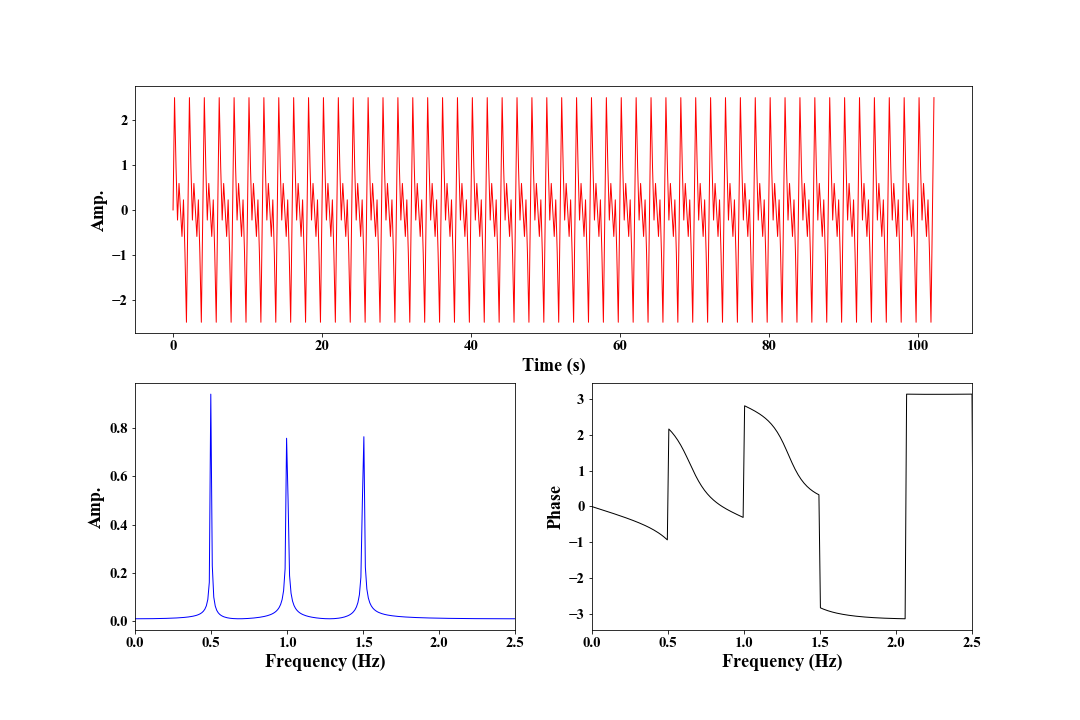### 1 Introduction

The $Fourier \ Transform$ convert the signal in one domain to another domain, for instance from time domain to frequency domain. The definition of $Fourier \ Transform$ is given by $$X(\omega) = \int_{-\infty}^\infty x(t)e^{-i \omega t} \tag{1}.$$

For the operation in programming, we need its discrete form, namely, $$X(k) = \sum_{n=0}^{N-1}x(n) e^{\frac{-i2\pi kn}{N}} \tag{2},$$ where, $k$ is the index of the $k_{th}$ frequency point, and we can find the $k_{th}$ frequency like this $$f_k = \frac{kf_s}{N} \tag{3},$$ $f_s$ is sampling rate of signal and $f_s=\frac{1}{\Delta t}$.

 1 2 3 4 5 6 7  import numpy as np n = 1024 x = np.random.randn(n) fft np.zeros_like(x, dtype=complex) xn = np.range(n) / n for k in range(n): fft[k] = sum( np.exp(-1j*2np.pi*k*xn) ) 

Actually, we have $$X(k) = X^*(N-k) \tag{4},$$ where, $^*$ means the complex conjugate. Here, we show how how eq. $(4)$ comes. \begin{aligned} X(N-k) &= \sum_{n=0}^{N-1}x(n)e^{-\frac{-i2\pi (N-k)n}{N}}\\ &= \sum_{n=0}^{N-1}x(n)e^{\frac{-i2\pi N}{N}}e^{\frac{i2\pi kn}{N}}\\ &= \sum_{n=0}^{N-1}x(n)e^{\frac{i2\pi kn}{N}}\\ &= X^*(k) \end{aligned} \tag{5}. Eq. $(4)$ suggests that we only need to compute the first half of $X(k), k=0, 1, 2, \cdots, \frac{N}{2}$.

### 2 FFT in Python

However, we just call the methods of libraries Numpy and Scipy in Python to implement $Fourier \ Transform$ as follows,

  1 2 3 4 5 6 7 8 9 10  import numpy as np from scipy import fft n = 1024 x = np.random.randn(n) # using fft of numpy. fft1 = np.ft.fft(x) # using fft of scipy. fft2 = fft.fft(x) 

### 3 Code demo

Here, we give an example to show how to convert a real signal in time domain to frequency domain.

  1 2 3 4 5 6 7 8 9 10 11 12 13 14 15 16 17 18 19 20 21 22 23 24 25 26 27 28 29 30 31 32 33 34 35 36 37 38 39 40 41 42 43 44  import numpy as np import matplotlib.pyplot as plt n = 512 dt = 0.2 fs = 1 / dt t = np.arange(n) * dt f = np.arange(n) * fs / n m = 3 # We generate a signal with frequencies (0.5, 1.0, 1.5). s = np.zeros(n) for i in range(m): s += np.sin(2*np.pi*(i+1)*0.5*t) # Get the FFT of s fd = np.fft.fft(s) / n * 2 # The amplitude spectrum. fa = abs(fd) # The phase spectrum. fp = np.arctan2(fd.imag, fd.real) plt.figure(figsize=(15, 10)) plt.subplot(211) plt.plot(t, s, 'r', lw=1) plt.xlabel('Time (s)', fontsize=18) plt.ylabel('Amp.', fontsize=18) plt.xticks(fontsize=15) plt.yticks(fontsize=15) plt.subplot(2, 2, 3) plt.plot(f, fa, 'b', lw=1) plt.xlim(0, fs/2) plt.xlabel('Frequency (Hz)', fontsize=18) plt.ylabel('Amp.', fontsize=18) plt.xticks(fontsize=15) plt.yticks(fontsize=15) plt.subplot(2, 2, 4) plt.plot(f, fp, 'k', lw=1) plt.xlim(0, fs/2) plt.xlabel('Frequency (Hz)', fontsize=18) plt.ylabel('Phase', fontsize=18) plt.xticks(fontsize=15) plt.yticks(fontsize=15) plt.show() 

The following figure shows the FFT of $x$.